# 不到 150 行代码写一个 Python 版的贪吃蛇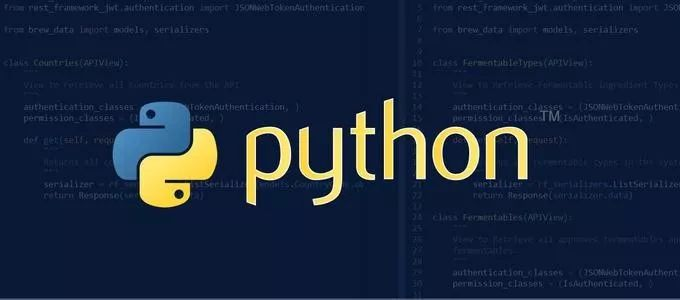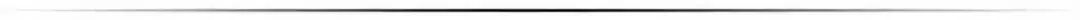pip install pygame


import pygame, sys
from pygame.locals import *


## Hello World

# 初始化
pygame.init()
# 新建窗口
screen = pygame.display.set_mode((640, 480))


# 设置窗口标题
pygame.display.set_caption("Hello World")
# 设置字体
font = pygame.font.Font(None, 30)
text = font.render('Hello World', True, pygame.Color("#FFFFFF"))


# 填充字体到画布
screen.blit(text, (100, 100))
# 刷新画布
pygame.display.update()


while 1:
for event in pygame.event.get():
if event.type == pygame.QUIT:
pygame.quit()
sys.exit()

screen.blit(text, (100, 100))
pygame.display.update()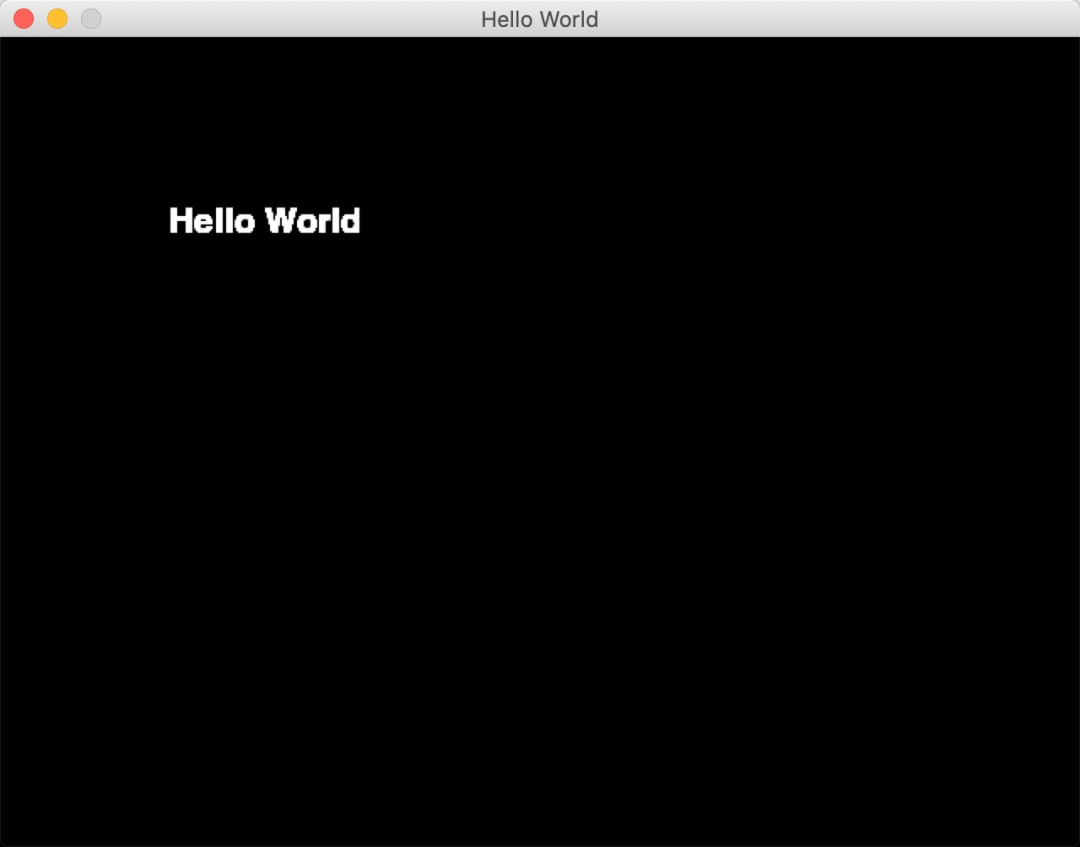## 贪吃蛇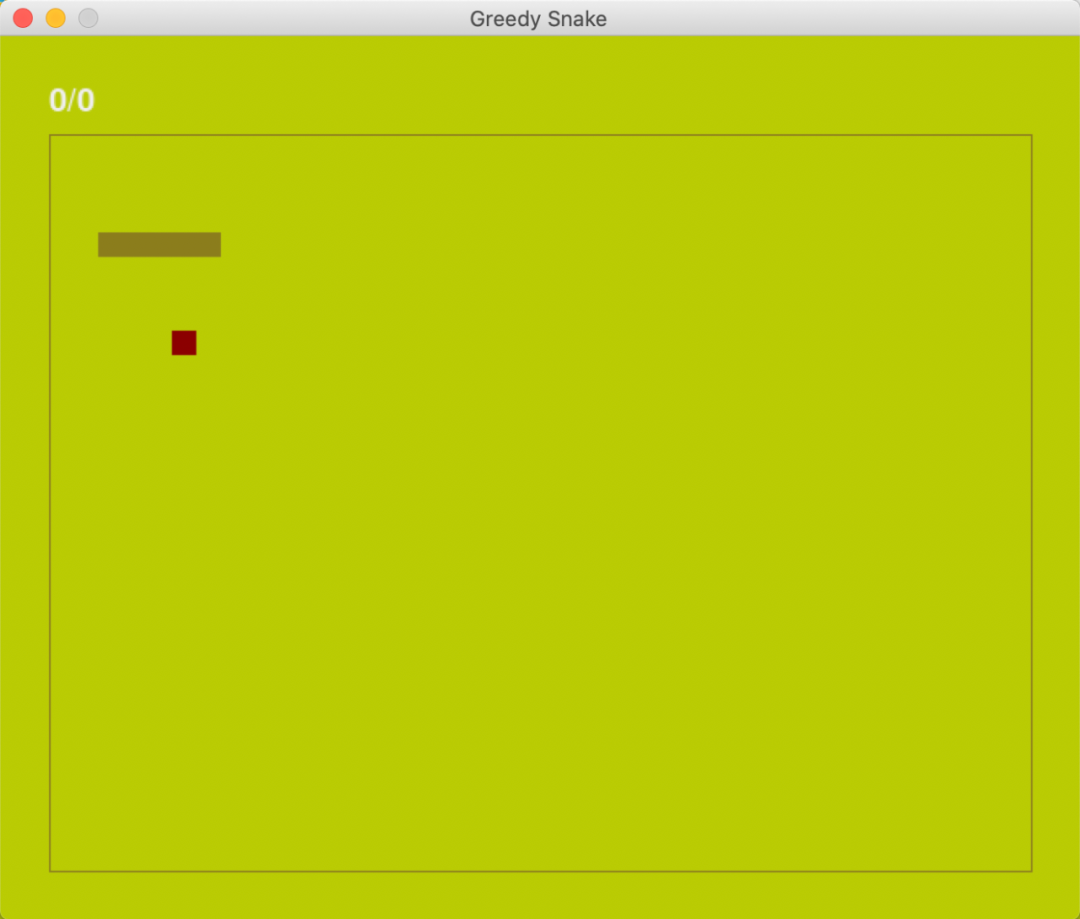### 颜色设置

# 颜色配置
snake_color = pygame.Color("#8B7D1C")
food_color = pygame.Color("#8B0000")
background_color = pygame.Color("#BACB03")
text_color = pygame.Color("#EFEFEF")


### 坐标设置

# 长度单位
pixel = 15
line = 44
row = 36
window_width = pixel * line
window_high = pixel * row

point_left_up = [pixel * 2, pixel * 4]
point_left_down = [pixel * 2, pixel * (row - 2)]
point_right_up = [pixel * (line - 2), pixel * 4]
point_right_down = [pixel * (line - 2), pixel * (row - 2)]


# 蛇头位置
snake_head = [pixel * 8, pixel * 8]
# 蛇 默认五个小方块
snake_body = [[snake_head - x * pixel, snake_head] for x in range(5)]


### 方向设置

# 方向
direction_right = 0
direction_up = 90
direction_left = 180
direction_down = 270


### 分数设置

# 分数设置
score = 5
filename = 'db.txt'

def write_score(content):
with open(filename, 'w+') as f:
f.write(str(content))

with open(filename, 'w+') as f:
return 0 if result.strip() == '' else int(result)


### 画蛇

# 显示文字
def display_message(text, color, size, postion):
font = pygame.font.Font(None, size)
text = font.render(text, True, color)
screen.blit(text, postion)
pygame.display.update()

# 画边线
def draw_box():
for point in [[point_left_up, point_right_up], [point_right_up, point_right_down],
[point_right_down, point_left_down], [point_left_down, point_left_up]]:
pygame.draw.line(screen, snake_color, point, point, 1)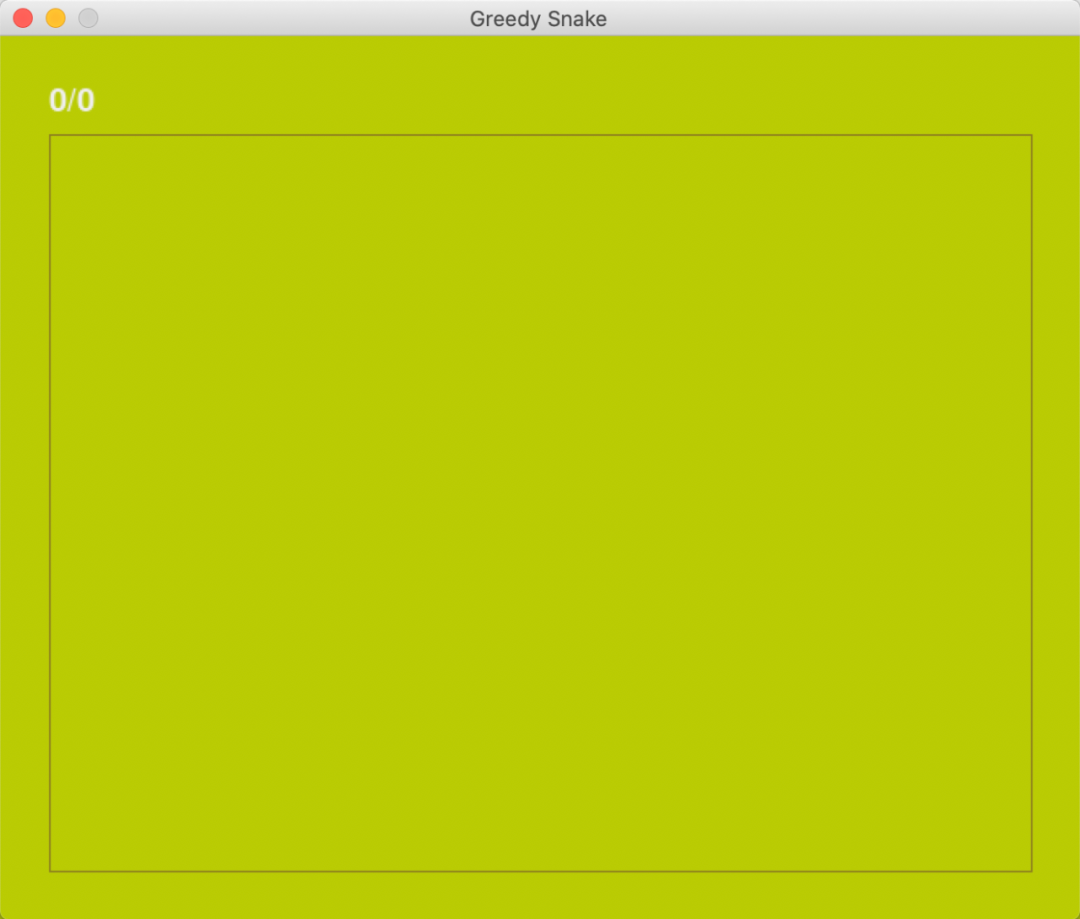# 随机产生食物
def create_food():
while True:
x = random.randint(point_left_up / pixel, point_right_down / pixel - 1) * pixel
y = random.randint(point_left_up / pixel, point_right_down / pixel - 1) * pixel
if [x, y] not in snake_body:
break
return [x, y]

def draw_snake(food_position):
# 画蛇
for point in snake_body:
pygame.draw.rect(screen, snake_color, Rect(point, point, pixel, pixel))
# 画食物
pygame.draw.rect(screen, food_color, Rect(food_position, food_position, pixel, pixel))### 让蛇动起来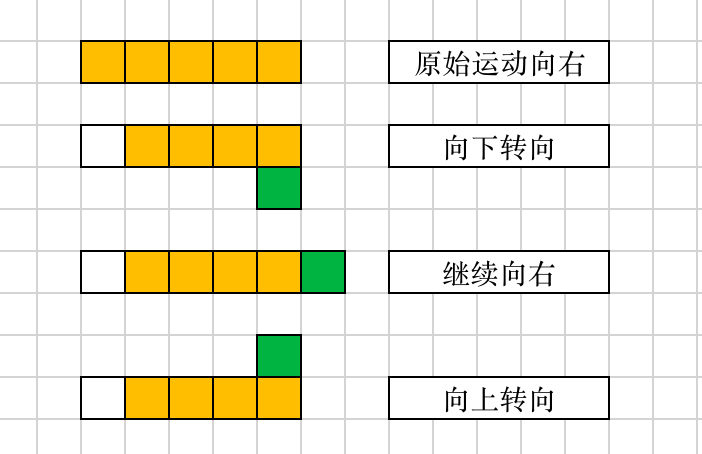move = {direction_right: [pixel, 0], direction_left: [-pixel, 0], direction_up: [0, -pixel], direction_down: [0, pixel]}
# 移动
# 移除蛇尾
snake_body.pop()


# 入口函数
def run():
food_position = create_food()
current_score = 0
origin_direction = direction_right
target_direction = origin_direction
while True:
# 监听键盘按键 退出 OR 换方向
for event in pygame.event.get():
if event.type == pygame.QUIT:
game_over(max_score, current_score)
if event.type == KEYDOWN:
# 箭头 OR asdw 控制方向
if event.key == K_RIGHT or event.key == K_d:
target_direction = direction_right
if event.key == K_LEFT or event.key == K_a:
target_direction = direction_left
if event.key == K_UP or event.key == K_w:
target_direction = direction_up
if event.key == K_DOWN or event.key == K_s:
target_direction = direction_down
# esc 退出
if event.key == K_ESCAPE:
game_over(max_score, current_score)
# 夹角为 90 or 270 可以转换方向
angle = abs(origin_direction - target_direction)
if angle == 90 or angle == 270:
origin_direction = target_direction

# 按 origin_direction 方向运动
# 吃到食物后重新生成
food_position = create_food()
current_score += score
else:
# 移除最后一格
snake_body.pop()
else:

# 画背景
screen.fill(background_color)
# 画边框
draw_box()
# 画蛇
draw_snake(food_position)
# 刷新画面
pygame.display.update()
# 更新分数
display_message(f"{current_score}/{max_score}", text_color, 30, (pixel * 2, pixel * 2))
display_message("Game Over", text_color, 50, (pixel * 16, pixel * 15))
# 控制游戏速度
time_clock.tick(speed)

if __name__ == '__main__':
run(


## 总结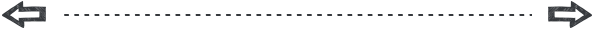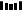11-27
08-0501-10
03-15
08-073519
10-182058
07-039223
01-314041
07-272158点击重新获取扫码支付余额充值